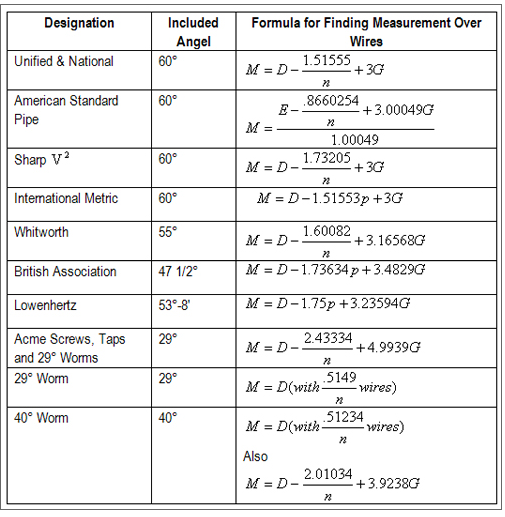## Why Osborn Products?

• ISO 9001:2015 Certified
• AS9100 Rev D Certified
• Aerospace Quality Gages
• Precision CNC Machining & Grinding
• Established in 1956# The Three Wire Method Of Measuring Pitch Diameter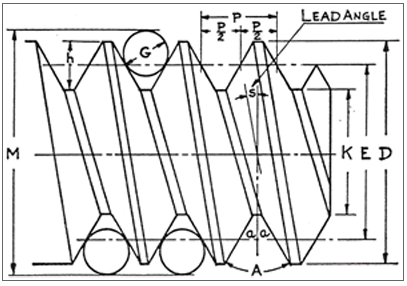In regards to the Three Wire Thread Measuring method, the following is an excerpt from page 35 of FED-STD-H28. "The accurate measurement of pitch diameter of a thread, which may be perfect as to form and lead, presents certain difficulties which result in some uncertainty as to its true value. The adoption of a standard uniform practice in making such measurements is, therefore, desirable in order to reduce such uncertainty of measurement to a minimum. The so-called "three  wire method" of measuring pitch diameter, as herein outlined, has been found to be the most generally satisfactory method when properly carried out, and is recommended for universal use in the direct measurement of thread plug and thread setting plug gages."

Important definitions and formulas:

1. ANGLE OF THREAD- This is the angle included between the sides of the thread measured in an axial plane. It is represented by the letter A. The half angle is represented by a small letter a. The angle of thread is known from the name of the thread. All Unified form and National threads have a 60° angle. Acme and some Worm threads have a 29° angle, and Whitworth threads have a 55° angle.
2. PITCH-This is the distance from a point on the screw thread to a corresponding point on the next thread measured parallel to the axis of the thread. It is represented by the letter p. (p=1/n).
• FED-STD-H28 uses the Greek letter alpha ? for ½ included angle of Thread (Axial Plain). In Appendix A, we use the English "a".
3. DEPTH OF THREAD- This is the distance from the crest to the root of the thread measured perpendicular to the axis of the screw or nut. ** It is represented by the letter h.
4. MAJOR DIAMETER- This is the largest diameter of the screw or nut. ** It is represented by the letter D. No formula is needed for the major diameter as it is used to identify the size of the screw. For instance a ¼  20 screw is one having a major diameter of 1/4 inch, and 20 thread per inch.
5. PITCH DIAMETER-The basic pitch diameter is the diameter where the thread thickness is equal to the space between the threads. If the flats at the top and bottom of the thread are the same, the pitch diameter will coincide with the middle of the sloping side of the thread. ** The pitch diameter is represented by the letter E.

6. Formula E = D  Depth of thread = D  h
Or E = D  Twice addendum

7. MINOR DIAMETER- This is the smallest diameter of the screw or nut. On the nut it corresponds to the tap drill size. ** It is represented by the letter K.

8. Formula K = D  2 X Depth of Thread = D -2h

9. LEAD ANGLE- This is the angle made by the pitch helix, with a plane perpendicular to the axis. ** It is represented by the letter s or.

10. Formula tan

NOTE: The reader is invited to refer to FED-STD-H28 and ANSI B1.7 for additional definitions having to do with elemental and dimensional information concerning screw threads.

11. BEST SIZE WIRES. * Wires which touch the thread at the pitch diameter are known as Best Size Wires. Such wires are used because the measurements of pitch diameter are least affected by errors that may be present in the angle of the thread. The diameter of the measuring wires is represented by the letter G.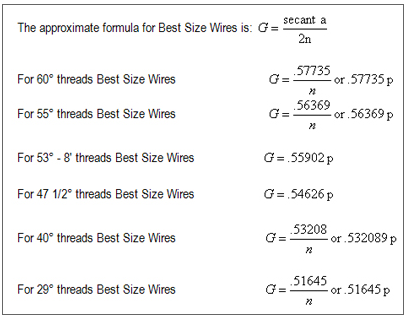Approximate Formulas for Measured Pitch Diameter

The following approximate formulas for computing the pitch diameter, from the measurement over wires, should be used only for screws having lead angles from 0° to 5°. These formulas neglect the effect of lead angle and give results which show the screw to be larger than the true condition. The formulas are for any wire size which will fit in the thread.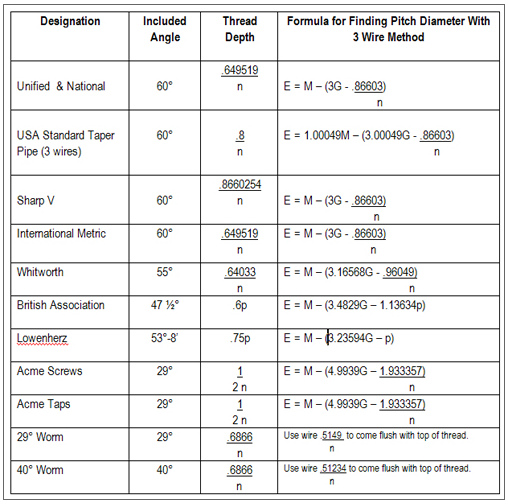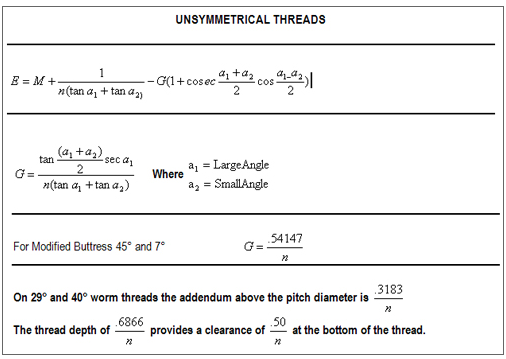Approximate Formulas for Basic Measurement Over Wires When cutting or grinding a screw thread, it is desirable to know what the measurement over wires would be for a screw of the theoretical basic size. The following approximate formulas are the same as those on the preceding page, but transposed and referred to the basic outside diameter of the screw. The same qualifications apply as were listed on the preceding page.